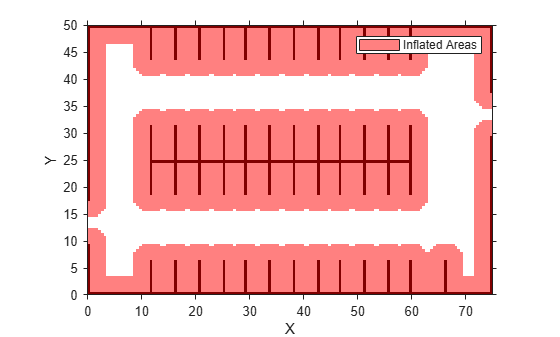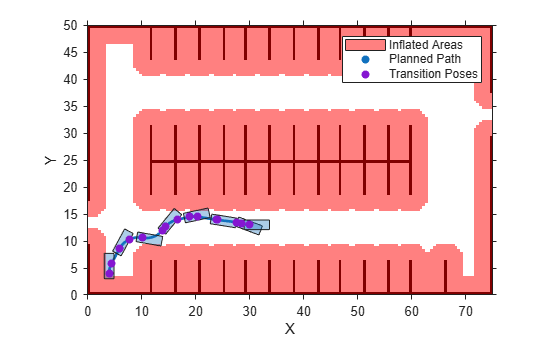# checkPathValidity

Check validity of planned vehicle path

## Syntax

``isValid = checkPathValidity(refPath,costmap)``
``isValid = checkPathValidity(refPoses,costmap)``

## Description

example

````isValid = checkPathValidity(refPath,costmap)` checks the validity of a planned vehicle path, `refPath`, against the vehicle costmap. Use this function to test if a path is valid within a changing environment.A path is valid if the following conditions are true: The path has at least one pose.The path is collision-free and within the limits of `costmap`. ```
````isValid = checkPathValidity(refPoses,costmap)` checks the validity of a sequence of vehicle poses, `refPoses`, against the vehicle costmap.```

## Examples

collapse all

Plan a vehicle path through a parking lot by using the optimal rapidly exploring random tree (RRT*) algorithm. Check that the path is valid, and then plot the transition poses along the path.

Load a costmap of a parking lot. Plot the costmap to see the parking lot and inflated areas for the vehicle to avoid.

```data = load('parkingLotCostmap.mat'); costmap = data.parkingLotCostmap; plot(costmap)```Define start and goal poses for the vehicle as [x, y, Θ] vectors. World units for the (x,y) locations are in meters. World units for the Θ orientation angles are in degrees.

```startPose = [4, 4, 90]; % [meters, meters, degrees] goalPose = [30, 13, 0];```

Use a `pathPlannerRRT` object to plan a path from the start pose to the goal pose.

```planner = pathPlannerRRT(costmap); refPath = plan(planner,startPose,goalPose);```

Check that the path is valid.

`isPathValid = checkPathValidity(refPath,costmap)`
```isPathValid = logical 1 ```

Interpolate the transition poses along the path.

`transitionPoses = interpolate(refPath);`

Plot the planned path and the transition poses on the costmap.

```hold on plot(refPath,'DisplayName','Planned Path') scatter(transitionPoses(:,1),transitionPoses(:,2),[],'filled', ... 'DisplayName','Transition Poses') hold off```## Input Arguments

collapse all

Planned vehicle path, specified as a `driving.Path` object.

Costmap used for collision checking, specified as a `vehicleCostmap` object.

Sequence of vehicle poses, specified as an m-by-3 matrix of [x, y, Θ] vectors. m is the number of specified poses.

x and y specify the location of the vehicle. These values must be in the same world units used by `costmap`.

Θ specifies the orientation angle of the vehicle in degrees.

## Output Arguments

collapse all

Indicates validity of the planned vehicle path, `refPath`, or the sequence of vehicle poses, `refPoses`, returned as a logical value of `1` or `0`.

A path or sequence of poses is valid (`1`) if the following conditions are true:

• The path or pose sequence has at least one pose.

• The path or pose sequence is collision-free and within the limits of `costmap`.

## Algorithms

To check if a vehicle path is valid, the `checkPathValidity` function discretizes the path. Then, the function checks that the poses at the discretized points are collision-free. The threshold for a collision-free pose depends on the resolution at which `checkPathValidity` discretizes.

## Version History

Introduced in R2018a